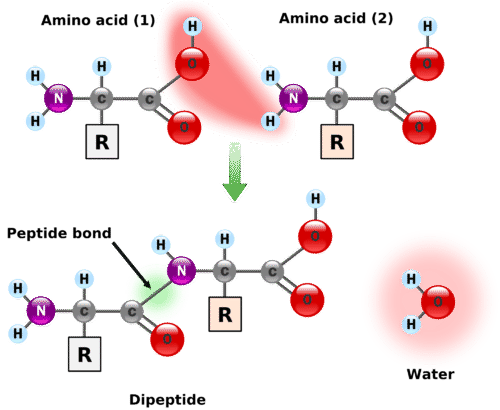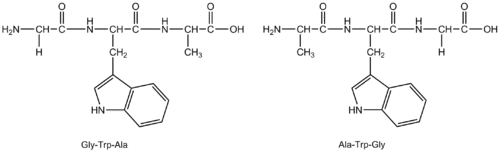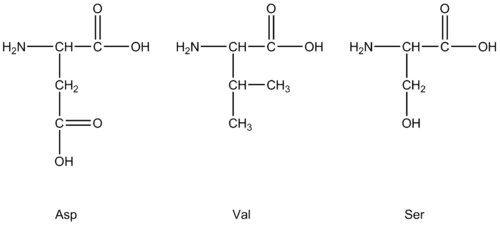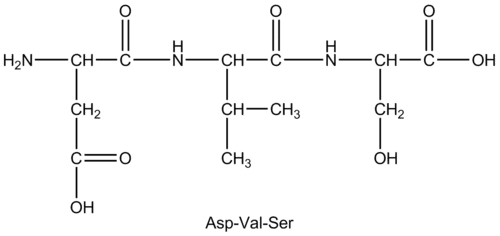# 13.2: Peptides

•• Contributed by Allison Soult
• Senior Lecturer (Chemistry) at University of Kentucky

Learning Outcomes

• Define polypeptide.
• Identify amide bond.
• Predict product of condensation of amino acids.
• Name polypeptides given the abbreviation of the amino acids.

Peptide cells in our bodies have an intricate mechanism for the manufacture of proteins. Humans have to use other techniques in order to synthesize the same proteins in a lab. The chemistry of peptide synthesis is complicated. Both active groups on an amino acid can react and the amino acid sequence must be a specific one in order for the protein to function. Robert Merrifield developed the first synthetic approach for making proteins in the lab, a manual approach which was lengthy and tedious (and, he won the Nobel Prize in Chemistry in 1984 for his work). Today, however, automated systems can crank out a peptide in a very short period of time.

## Peptides

A peptide is a combination of amino acids in which the amine group of one amino acid has undergone a reaction with the carboxyl group of another amino acid. The reaction is a condensation reaction, forming an amide group $$\left( \ce{CO-N} \right)$$, shown below.Figure $$\PageIndex{1}$$: Amino acids undergo condensation to form a molecule called a dipeptide. The $$\ce{C-N}$$ bond is called a peptide bond.

A peptide bond is the amide bond that occurs between the amine nitrogen of one amino acid and the carboxyl carbon of another amino acid. The resulting molecule is called a dipeptide. Notice that the particular side chains of each amino acid are irrelevant since the $$\ce{R}$$ groups are not involved in the peptide bond.

The dipeptide has a free amine group on one end of the molecule (known as the $$\ce{N}$$-terminus) and a free carboxyl group on the other end (known as the $$\ce{C}$$-terminus). Each is capable of extending the chain through the formation of another peptide bond. The particular sequence of amino acids in a longer chain is called an amino acid sequence. By convention, the amino acid sequence is listed in the order such that the free amine group is on the left end of the molecule and the free carboxyl group is on the right end of the molecule. For example, suppose that a sequence of the amino acids glycine, tryptophan, and alanine is formed with the free amine group as part of the glycine and the free carboxyl group as part of the alanine. The amino acid sequence can be easily written using the abbreviations as Gly-Trp-Ala. This is a different sequence from Ala-Trp-Gly because the free amine and carboxyl groups would be on different amino acids in that case.Example $$\PageIndex{1}$$

Draw the polypeptide Asp-Val-Ser.

Solution

1. Identify the structures of each of the three given amino acids and draw them in the same order as given in the name.2. Leaving the order the same, connect the amino acids to one another by forming peptide bonds. Note that the order given in the name is the same way the amino acids are connected in the molecule. The first one listed is always the $$\ce{N}$$-terminus of the polypeptide.Example $$\PageIndex{2}$$

List all of the possible polypeptides that can be formed from cysteine (Cys), leucine (Leu), and arginine (Arg).

Solution

Although there are only three amino acids, the order in which they are bonded changes the identity, properties, and function of the resulting polypeptide. There are six possible polypeptides formed from these three amino acids.

Cys-Leu-Arg

Cys-Arg-Leu

Leu-Cys-Arg

Leu-Arg-Cys

Arg-Cys-Leu

Arg-Leu-Cys